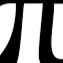# Unresolved Detail In Plotted Equations

Sometimes the calculator detects that an equation is too complicated to plot perfectly in a reasonable amount of time. When this happens, the equation is plotted at lower resolution.

Was this article helpful?
221 out of 246 found this helpful
Have more questions? Submit a request

#### 927 Comments

•BraynBeats

\frac{\frac{\sin x^3}{\tan x^2}}{y}=\tan y^2

•Jmanmeneer

This one is actually really majestic.

\frac{\operatorname{floor}\left(\frac{\frac{\left(x^y\right)}{\operatorname{floor}\left(y^x\right)}}{\operatorname{mod}\left(2^y,20\right)}\right)}{y^x}=\frac{\operatorname{floor}\left(\frac{\frac{\left(y^x\right)}{\operatorname{floor}\left(x^y\right)}}{\operatorname{mod}\left(2^x,20\right)}\right)}{x^y}

•Kerndr

sin(xy)=0 is pretty intense

•Kerndr

and sin(xy)=sin(y)+sin(x) is cool

•Creatorext

try \operatorname{floor}\left(yx\right)=xy^2

•Thurston Domina

\operatorname{floor}\left(xy\right)-\left(xy\right)!=-1

•Skilletrocks424

x!!!=\tan\left(y\right)! works too

•70654

Try this:

sin(x^y)=y^sin(x)

•70654

it totaly glitches desmos

•70654

also try x^y=sin(x^2)

it doesn't bring up the message, but it looks really cool.

•GlitchedGraph666

\sin\left(\operatorname{floor}\left(\frac{x}{y^2}\right)\sin\left(\frac{\operatorname{floor}\left(x^2\right)}{\sin\left(x^{\frac{y}{2}}\right)}\right)\right)\cos\left(\sqrt{x\cdot\sin\left(y^2\right)}\right)=\cos\left(x^y\right)

•GlitchedGraph666

y\cdot x^{\sin\left(y\right)}+x\cdot\cos\left(x^y+\sin\left(x^y\right)\right)=\sin\left(x^y\right)

•Jacob Pe2960

20 messy equations on one graph.

•mATT C
•Ritterayfoh00

simple, but it gives the message.

\frac{y}{x}=x^{y^x}

•Gabriel Bugg

x^y\csc\left(y^x\right)=\frac{\left(x^y\sec\left(y^x\right)\right)^{xy}}{9}-\frac{\left(y^x\sec\left(x^y\right)\right)^{xy}}{9}

•Youngcol000

\cos\left(y+x\right)=\sin\left(y^5+x\right)

THish here is a good one

•Psychomaniac14

My favourite example is sin(cos(tan(x)))=2(y^2)(x^2)+csc(y^2)

•Psychomaniac14

1=\left|\sin\left(xy\right)\right|

it refuses to even show!

•Psychomaniac14

\sin\left(\cos\left(\tan\left(\csc\left(\sec\left(\cot\left(xy\right)\right)\right)\right)\right)\right)=1

another one that won't load

•mATT C

y\le\operatorname{round}\left(\left(\sum_{n=1}^{\operatorname{round}\left(\left|\frac{0}{y}+x\sin\left(\prod_{a=1}^{\left|x\right|+1}a\left(-1\right)^a\sin a\right)\right|+1\right)}\frac{n\sin\left(n\right)^n}{2}\right)\right)

Zoom out three times

Now that hurts

•GD TheProGamer711

cos(x)=-1 will show up for a sec then just vanish and never reappear...

•Gavmanno (Gavmanno)

This is sure interesting

\frac{\sqrt{x^2+y^2}\tan\left(x^2+y^2\right)}{4}=\sin\left(y\right)

•25jw0157

I thank your some of your functions and equations to create this: https://www.desmos.com/calculator/frfgddorer

•GlitchedGraph666

xy\pi\sin\left(x^y\right)+\cos\left(x\cdot2\right)=\tan\left(x^2\right)+x^2\cdot\sin\left(\sqrt{\pi^{\cos\left(x\cdot y\right)}}\right)

•GlitchedGraph666

x\cdot\cos\left(y+x^y\right)\pi+\sin\left(x\cdot y^{\cos\left(x\cdot666\right)}\right)=\cos\left(x+y\right)

•Aboldi19

Impossible: \frac{1}{2}<\operatorname{floor}\left(\operatorname{mod}\left(\operatorname{floor}\left(\frac{y}{17}\cdot2^{\left(-17\cdot\operatorname{floor}\left(x\right)-\operatorname{mod}\left(\operatorname{floor}\left(y\right),17\right)\right)}\right),2\right)\right)

•Xinli Dai the Pi Guy
•First Last

Here are two:

1. csc(x)=x
2. sec(x)=x
Edited by First Last
•First Last

The graphing calculator can graph fine detail for the equation 1=nCr(x,y) but it can't

1=nCr(|x|,y)
1=nCr(x,|y|)

or

1=nCr(|x|,|y|)

Edited by First Last
Article is closed for comments.